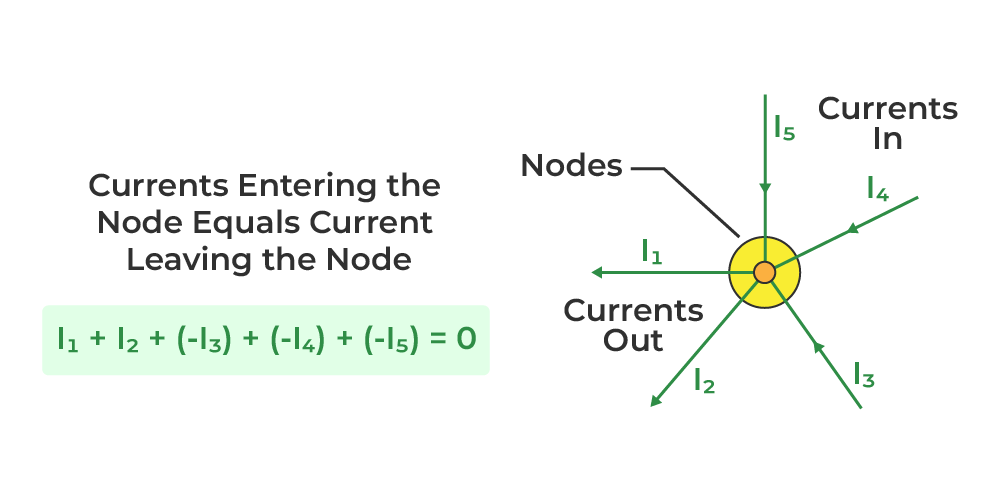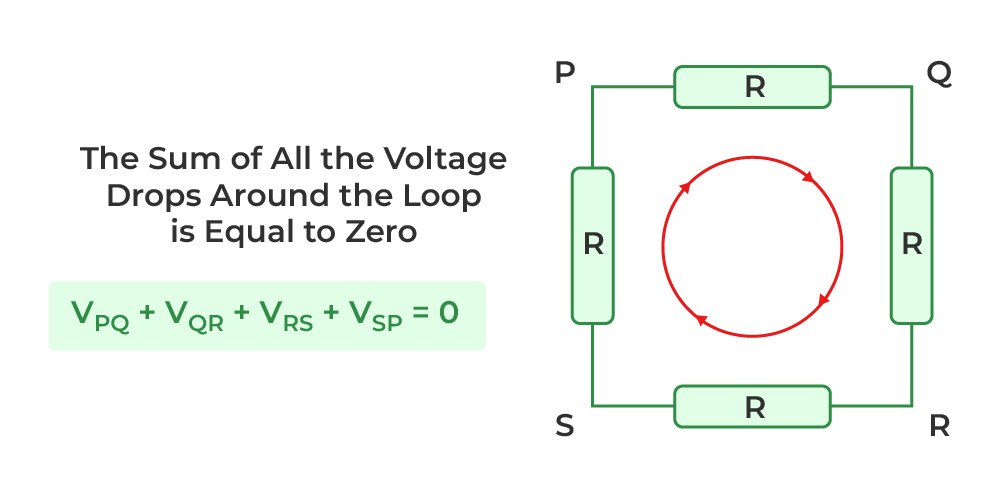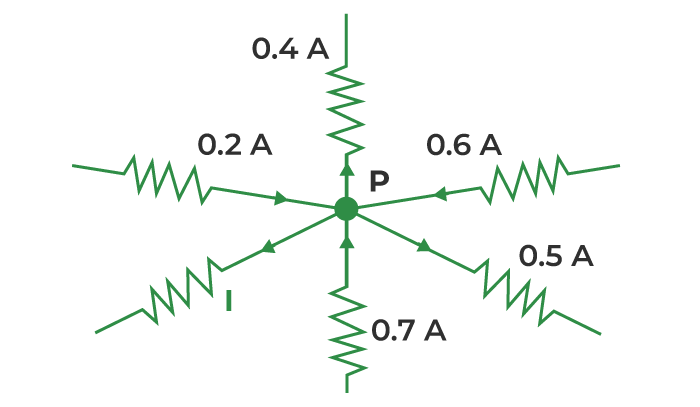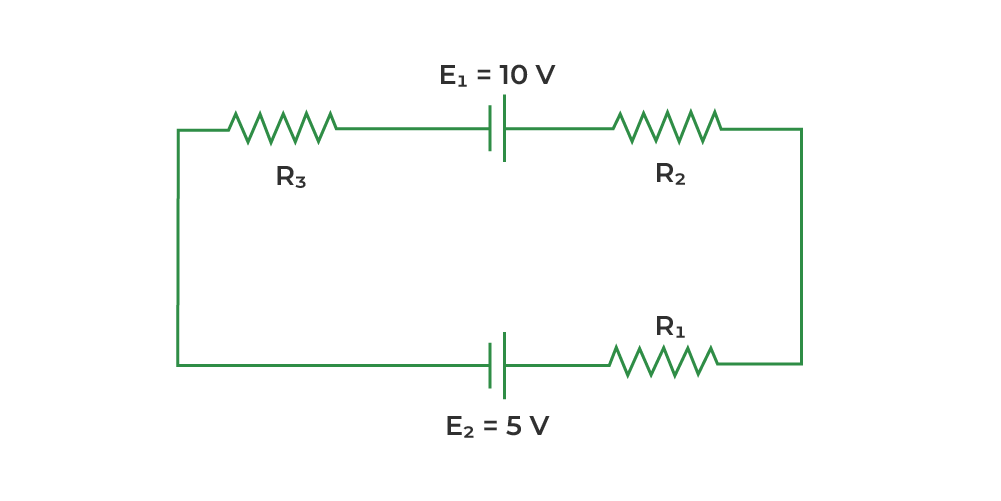GFG App
Open AppBrowser
Continue

Kirchhoff’s Law is one of the basic laws which is used in electrostatics to solve complex circuit questions. Kirchhoff’s Law is given by Gustav Robert Kirchhoff who is a famous German physicist he gave us two laws Kirchhoff’s Current Law and Kirchhoff’s Voltage Law which are discussed in this article.

## History of Gustav Robert Kirchhoff

Gustav Robert Kirchhoff was a Russian-born German physicist, his job was investigating electrical conduction. Kirchhoff’s Circuit Law is the combination of Kirchhoff’s Voltage Law (KVL) and Kirchhoff’s Current Law (KCL) which were published in 1845. Circuit analysis is carried out using these laws. They are helpful in the calculation of current flow and voltage flow in various streams across the network.

## What are Kirchhoff’s Laws?

Kirchhoff’s Laws are the basic law used in circuit analysis to solve complex circuit problems. Gustav Robert Kirchhoff gave us two laws Kirchhoff’s Voltage Law (KVL) and Kirchhoff’s Current Law (KCL) which are widely used in circuit analysis. Kirchhoff’s Voltage Law (KVL) is similar to the law of conservation of energy, whereas Kirchhoff’s Current Law (KCL) is similar to the law of conservation of charge.

## Kirchhoff’s Current Law (Kirchhoff’s I Law )

This law says that “the total current or charge entering a junction or node is precisely identical to the total current or charge exiting the node, as no charge is lost at the node.” To put it another way, the algebraic total of all currents entering and exiting a node must be zero. Kirchhoff’s Current Law (KCL) is also known as Kirchhoff’s I Law.

Note:

Kirchhoff’s (I) law is similar to the Law of Conservation of charge. As a result, a Nord or junction is a point in a circuit that does not serve as a charge source or sink.

Therefore,

nk=1 IK = 0

Where n denotes the total number of branches at the node with currents flowing toward or away from it.

i.e.

I(exiting)+I(entering) = 0

For Example,

From a resistor network, concentrate on node A. This node is related to five branches. The three incoming currents, i3, i4 and i5 and the two incoming currents, i1 and i2. The sum of total incoming and outgoing currents at node A will now equal zero, according to Kirchhoff’s current law.Consider the two currents that enter the node, i1, and i2, as positive, and the two currents that leave the node, i3 and i4, as negative.

i1+i2–i3–i4=0

## Kirchhoff’s Voltage Law (Kirchhoff’s II Law)

The voltage drop around a loop equals the algebraic total of the voltage drop across every electrical component linked in the same loop for any closed network and also equals zero, Kirchhoff’s Voltage Law (KVL) is also known as Kirchhoff’s II Law.

Notes:

Kirchhoff’s (II) law is similar to the Law of Conservation of energy. Because the net change in the energy of a charge after it completes a closed route must be zero.

For Example,

Consider a section of a resistor network with an internal closed loop, as seen in the diagram given below. In the closed loop, we wish to write the voltage change. The total of all voltage drops between the components linked in the loop PQRSP is zero, according to Kirchhoff’s voltage law.So,

nk=1 Vk=0

The total number of electrical components in the loop is given by n.

i.e.

VPQ+VQR+VRS+VSP=0

## Applying Kirchhoff’s Laws

When using KCL, the currents exiting a junction must be considered negative, while the currents entering the junction must be considered positive.

Also, while using KVL, we keep the same anti-clockwise or clockwise orientation from the beginning of the loop and account for all voltage decreases as negative and increases as positive. This brings us back to the initial point when the total voltage loss is zero.

## Sign Conventions

• In a loop, a rise in potential difference or EMF from lower to higher is always seen as positive.
• In a loop, a reduction in potential difference or EMF from higher to lower is always seen as negative.
• If the looping direction is the same as the current flowing through the circuit, the voltage drop across the resistor is considered negative.

## Uses of Kirchhoff’s Law

• It is used to find out how much current is flowing and how much voltage is dropped in various areas of the complicated circuit.
• It aids in determining the direction of current in various circuit loops.
• Kirchhoff’s Laws can help you comprehend how energy moves via an electric circuit.

## Limitations of Kirchhoff’s Laws

There are various limitations associated with Kirchhoff’s Laws which are:

• Kirchhoff’s law has one fundamental flaw: it implies that there is no changing magnetic field throughout the loop’s region, which might induce a change in magnetic flux and the creation of EMF in the circuit. This might result in a calculation mistake for high-frequency AC circuits.
• Kirchhoff also ignored the impact of the electric field generated by other circuit components.
• KCL works with the premise that current only travels through conductors and wires. While parasitic capacitance can no longer be overlooked in High-Frequency circuits. Because conductors or wires are serving as transmission lines in certain circumstances, electricity may start flowing in an open circuit.

## Kirchhoff’s Law Solved Examples

Example 1: Find the value of I from the circuit in the figure below.Solution:

Apply Kirchhoff’s first law to the point P in the supplied circuit.

Consider the sign convention: positive arrows point toward P, whereas negative arrows point away from P.

As a result, we now have the following:

0.2 A – 0.4 A + 0.6 A – 0.5 A + 0.7 A – I = 0

1.5 A – 0.9 A – I = 0

0.6 A – I = 0

I = 0.6A

Example 2: Find the value of the current passing through the resistance R1 = 10 Ω in the circuit below using Kirchhoff’s principles, when R2 and R3 both are equal and their value is 5 Ω.Solution:

Applying Kirchhoff’s Law in the above circuit, Let’s take the current ‘i’ passing through the loop in the clockwise direction

-10 + 5(i) + 5(i) + 10(i) + 5 = 0

20i -5 = 0

20i = 5

i = 5 / 20 =1/4

The current passing through R1 is 1/4 amp.

Also, Check

## FAQs on Kirchhoff’s law

### Question 1: Who discovers Kirchhoff’s law?

Kirchhoff’s law was discovered by a german physicist, Gustav Robert Kirchhoff

### Question 2: What is Kirchhoff’s Voltage Law?

Kirchhoff’s II Law also called Kirchhoff’s Voltage Law states that ” For any closed network Voltage around a loop is equal to the sum of voltage drop in the same loop  and it is equal to zero.”

### Question 3: What is Kirchhoff’s Current Law?

Kirchhoff’s I Law also called Kirchhoff’s Current Law states that ” Total current entering in a junction is equal to the total current leaving that junction.”

### Question 4: Kirchhoff’s second law is also known as?

Kirchhoff’s second law is also known as  Kirchhoff’s Voltage Law.

### Question 5: How many Kirchhoff laws are there?

There are two Kirchhoff laws

• Kirchhoff’s First Law or Kirchhoff’s Current Law
• Kirchhoff’s Second Law or Kirchhoff’s Voltage Law